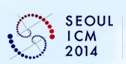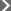SEOUL ICM 2014 International Congress of Mathematicians
 August 13 - 21, 2014 Coex , Seoul , Korea
 ProgramScientific Program
Home > Program > Scientific Program

Scientific Program

The
IMU Executive Committee has appointed the ICM 2014 Program Committee (PC) at its meeting in February 2011. The chair of the ICM 2014 PC is Carlos Kenig, (PC-Chair-ICM2014@mathunion.org), the Louis Block Distinguished Service Professor of the University of Chicago, and the PC has set up the structure of the scientific program of ICM 2014. All Plenary and Invited Lectures will be published in the Proceedings after the Congress, and Abstracts of all lectures and of all short presentations will be distributed free of charge to registered participant at Congress check-in.

As in previous years at the ICM, ICM 2014 will offer a wide-ranging, attractive scientific program consisting of plenary and invited lectures, short communications, poster sessions and other scientific activities.

The following subject areas have been chosen for ICM 2014 (section descriptions as well as the number of plenary and invited lectures to be given in each section):

ICM Proceedings Style Files
In order to maintain consistency of the style in the ICM Proceedings, invited plenary and sectional speakers are encouraged to process their electronic files using the style files of the ICM Proceedings. All necessary files, including examples, can be downloaded here.

Click the sections to view invited speakers and panels list.

Model theory. Set theory. Recursion theory. Proof theory. Applications.
Connections with sections 2, 3, 13, 14.

Groups (finite, infinite, algebraic) and their representations. Rings, Algebras and Modules (except as specified in other sections, Geometry, or Lie theory). Algebraic K-theory, Category theory, Computational aspect of algebra and applications.
Connections with sections 1, 3, 4, 5, 6, 7, 13, 14.

Analytic and algebraic number theory. Local and global fields and their Galois groups. Zeta and L-functions. Diophantine equations. Arithmetic on algebraic varieties. Diophantine approximation, transcendental number theory, and geometry of numbers. Modular and automorphic forms, modular curves, and Shimura varieties. Langlands program. p-adic analysis. Number theory and physics. Computational number theory and applications, notably to cryptography.
Connections with sections 1, 2, 4, 7, 11, 12, 13, 14.

Algebraic varieties, their cycles, cohomologies, and motives. Schemes. Geometric aspects of commutative algebra. Arithmetic geometry. Rational points. Low-dimensional varieties. Singularities and classification. Birational geometry. Moduli spaces and enumerative geometry. Derived categories. Abelian varieties. Transcendental methods, topology of algebraic varieties. Complex differential geometry, Kähler manifolds and Hodge theory. Relations with mathematical physics and representation theory. Real algebraic and analytic sets. Rigid and p-adic analytic spaces. Tropical geometry. Non-commutative geometry.
Connections with sections 2, 3, 5, 6, 7, 8, 11, 13, 14.

Local and global differential geometry. Non-linear and fully non-linear geometric PDE. Geometric flows. Geometric structures on manifolds. Riemannian and metric geometry. Geometric aspects of group theory. Conformal geometry, Kähler geometry, Symplectic and Contact geometry, Geometric rigidity, General Relativity.
Connections with sections 2, 4, 6, 7, 8, 9, 10, 11, 12, 16, 17.

Algebraic Topology, Differential Topology, Geometric Topology, Floer and gauge theories, Low-dimensional manifolds including knot theory and connections with Kleinian groups and Teichmüller theory, Symplectic Geometry and contact manifolds, and Topological quantum field theories.
Connections with sections 2, 4, 5, 7, 8, 11.

Algebraic and arithmetic groups. Structure, geometry, and representations of Lie groups and Lie algebras. Related geometric and algebraic objects, e.g. symmetric spaces, buildings, vertex operator algebras, quantum groups. Non-commutative harmonic analysis. Geometric methods in representation theory. Discrete subgroups of Lie groups. Lie groups and dynamics, including applications to number theory.
Connections with sections 2, 3, 4, 5, 6, 8, 9, 11, 12, 13.

Classical analysis. Real and Complex analysis in one and several variables, potential theory, quasiconformal mappings. Harmonic analysis. Linear and non-linear functional analysis, operator algebras, Banach algebras, Banach spaces. Non-commutative geometry, spectra of random matrices. Asymptotic geometric analysis. Metric geometry and applications. Geometric measure theory.
Connections with sections 5, 6, 7, 9, 10, 11, 12, 13, 14, 15, 16.

Topological and symbolic dynamics. Geometric and qualitative theory of ODE and smooth dynamical systems, bifurcations and singularities. Hamiltonian systems and dynamical systems of geometric origin. One-dimensional and holomorphic dynamics. Strange attractors and chaotic dynamics. Multidimensional actions and rigidity in dynamics. Ergodic theory including applications to combinatorics and combinatorial number theory. Infinite dimensional dynamical systems and PDE.
Connections with sections 5, 7, 8, 10, 11, 12, 13, 15, 16.

Solvability, regularity, stability and other qualitative properties of linear and non-linear equations and systems. Asymptotics. Spectral theory, scattering, inverse problems. Variational methods and calculus of variations. Geometric Evolution equations. Optimal transportation. Homogenization and multiscale problems. Relations to continuous media and control. Modeling through PDEs.
Connections with sections 5, 8, 9, 11, 12, 15, 16, 17.

Quantum mechanics. Quantum field theory including gauge theories. General relativity. Statistical mechanics and random media. Integrable systems. Supersymmetric theories. String theory. Fluid dynamics.
Connections with sections 4, 5, 6, 7, 8, 9, 10, 12.

Stochastic processes, Interacting particle systems, Random media, Random matrices, conformally invariant models, Stochastic networks, Stochastic geometry, Statistical inference, High-dimensional data analysis, Spatial methods.
Connections with sections 3, 5, 7, 8, 9, 10, 11, 13, 14, 15, 16, 17

Combinatorial structures. Enumeration: exact and asymptotic. Graph theory. Probabilistic and extremal combinatorics. Designs and finite geometries. Relations with linear algebra, representation theory and commutative algebra. Topological and analytical techniques in combinatorics. Combinatorial geometry. Combinatorial number theory. Additive combinatorics. Polyhedral combinatorics and combinatorial optimization.
Connections with sections 1, 2, 3, 4, 7, 9, 12, 14.

Complexity theory and design and analysis of algorithms. Formal languages. Computational learning. Algorithmic game theory. Cryptography. Coding theory. Semantics and verification of programs. Symbolic computation. Quantum computing. Computational geometry, computer vision.
Connections with sections 1, 2, 3, 4, 12, 13, 15.

Design of numerical algorithms and analysis of their accuracy, stability, and complexity. Approximation theory. Applied and computational aspects of harmonic analysis. Numerical solution of algebraic, functional, stochastic, differential, and integral equations. Grid generation and adaptivity.
Connections with sections 8, 9, 10, 12, 14, 16, 17.

Minimization problems. Controllability, observability, stability. Robotics. Stochastic systems and control. Optimal control. Optimal design, shape design. Linear, non-linear, integer, and stochastic programming. Applications.
Connections with sections 9, 10, 12, 15, 17.

Mathematics applied to the physical sciences, engineering sciences, life sciences, social and economic sciences, and technology. Bioinformatics. Mathematics in interdisciplinary research. The interplay of mathematical modeling, mathematical analysis, and scientific computation, and its impact on the understanding of scientific phenomena and on the solution of real life problems.
Connections with sections 9, 10, 11, 12, 14, 15, 16.

All aspects of mathematics education, from elementary school to higher education. Mathematical literacy and popularization of mathematics.

Historical studies of all of the mathematical sciences in all periods and cultural settings.

 Copyrights ⓒ 2010-2015 International Congress of Mathematicians 2014 All right Reserved. The Korea Science and Technology Center 710 New Bldg., 635-4 Yeoksam-dong, Gangnam-gu, Seoul 135-703, Republic of Korea Tel: +82-2-563-2014 Fax: +82-2-563-2022 E-mail: icm@icm2014.org Homepage: www.icm2014.org